# Go tutorial: Build a card game with Golang

Sep 09, 2021 - 9 min readErin Schaffer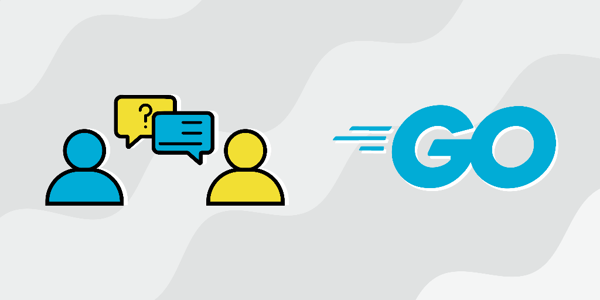Golang, or Go, is a popular programming language used by many top tech companies. If you dream of working for one of these top companies, it’s important to have experience with the programming languages they use. The best way to learn any programming language is to dive right in and get real-world, hands-on experience. A solid portfolio with coding projects can help demonstrate your skills and experience in a programming language. Today, we’re going to walk you through a real-world Go portfolio project.

Let’s get started!

We’ll cover:

## Project description

Card games are popular all around the world, with people of all ages playing different variations of them. Let’s say that you work for a startup that wants to create a web app to play different card games. They want to design common games, like poker and solitaire, as well as create their own unique games. All of the games can be played in single-player or multiplayer mode. Since there will be a single-player option, your team needs to create an “opponent” to play against users.

The first part of the project involves implementing a feature that helps the user play a variation of poker. You will have to design the feature so that it can determine if a given hand of cards is a hand of straights or not. The second part of the project involves creating a feature for a custom card game named Fizzle. You will have to design the feature so that the user can find the maximum points that can be obtained by picking out cards from a set of ten random cards.

### Features

• Feature 1: Determine if a hand of straights is possible.
• Feature 2: Find the maximum number of points that can be obtained from a set of ten random cards. Now that you know the background, let’s get started working on the first feature!

## Building feature 1

For feature #1, we’re working on a poker game variation concerned with a hand of straights. In traditional poker, players have sets of five cards (called hands). Normally, a hand of straights is formed by five cards of sequential ranks, like 9, 8, 7, 6, and 5.

In our new variation of poker, a number k will be determined by a dice roll. If the dice roll a 1, it should be rolled again. That way, k will always be in the range of 2-6. A hand of straights is only possible if k sets of cards can be formed using all of the cards in the given hand.

### Example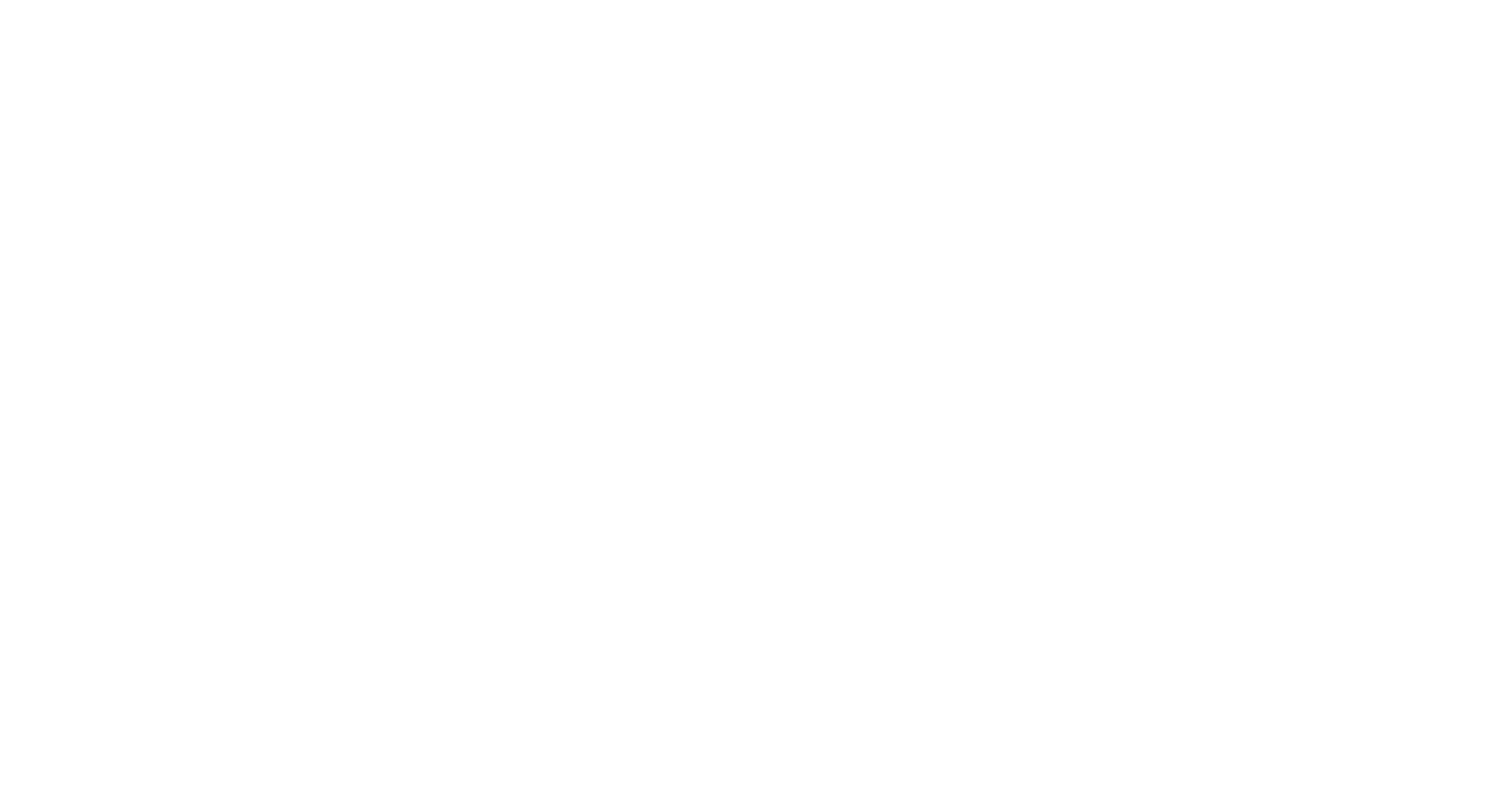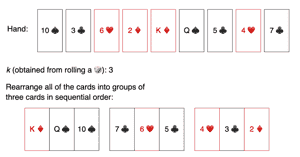In the above example, we can see that we were dealt a hand of nine cards. The dice rolled a 3, so k is 3. Then, the cards were arranged into three groups. Each group contains three cards in sequential order. During implementation, we’ll receive these cards in an array, like {10, 3, 6, 2, 13, 12, 5, 4, 7}. The jack, king, and queen cards are denoted by 11, 12, and 13 respectively. The number after the dice roll is given as an integer. The module should return true if a hand of straights can be formed. If a hand of straights can’t be formed, it should return false.

### Solution

The common intuition behind the solution is to try and form groups of size k, starting with the lowest card. Once the lowest card is identified, a hand of straights is only possible if the lowest card is at the bottom end of a k-sized group. For example, if k is 4 and the lowest card is 2, we know that the group will be 2, 3, 4, 5. If we can’t find this group, the hand isn’t a hand of straights.

Let’s get started coding the feature! We’ll break the solution down into steps. Before we get started, we need to import the proper packages so we can test our code at the end.

package main
import (
"fmt"
"sort"
)

Step 1: Set up the function.

func isHandOfStraights(hand []int, k int) bool{

Step 2: Check if the number of cards in the hand is divisible by k. If not, we can’t create groups, so return false.

    if len(hand) % k != 0{
return false
}

Step 3: Count the occurrences of each card in the given hand.

    count := make(map[int]int)
for _, i := range hand{
count[i] = count[i] + 1
}

Step 4: Sort the list and start traversing it from the lowest-ranking card. We can use a hash map by storing card numbers as keys and occurrences as values.

    sort.Ints(hand)
i := 0
n := len(hand)

Step 5: Use a nested loop that runs k times.

        for i < n {
current := hand[i]
for j := 0; j < k; j++ {

Step 5.1: Check if the current card and the next k-1 cards (in increasing ranking) are in the count map. If any of them don’t exist, return false.

            if _, ok := count[current + j]; !ok || count[current + j] == 0 {
return false
}

Step 5.2: When each of the required cards is found, decrease its number of occurrences in the count.

            count[current + j]--
}

Step 5.3: After a complete group is found, use a while loop to find the next group’s smallest card and determine which of the next cards in count has more than zero occurrences left.

        for i < n && count[hand[i]] == 0{
i++
}
}

Step 6: Return true if all cards are sorted into groups.

   return true
}

Now, let’s test our feature using two sample hands.

package main
import (
"fmt"
"sort"
)
func isHandOfStraights(hand []int, k int) bool{
if len(hand) % k != 0{
return false
}

count := make(map[int]int)
for _, i := range hand{
count[i] = count[i] + 1
}

sort.Ints(hand)
i := 0
n := len(hand)

for i < n {
current := hand[i]
for j := 0; j < k; j++ {
if _, ok := count[current + j]; !ok || count[current + j] == 0 {
return false
}
count[current + j]--
}
for i < n && count[hand[i]] == 0{
i++
}
}
return true
}

func main() {
hand := []int{5,2,4,4,1,3,5,6,3}
k := 3
fmt.Println(isHandOfStraights(hand, k))

hand2 := []int{1,9,3,5,7,4,2,9,11}
k = 2
fmt.Println(isHandOfStraights(hand2, k))
}

Start building a Go project portfolio with our 1-week Educative Unlimited Trial. Educative’s text-based courses are easy to skim and feature live coding environments, making learning quick and efficient.

Decode the Coding Interview in Go: Real-World Examples

## Building feature 2

For feature #2, we’re working on a custom card game called Fizzle. In this game, the dealer shuffles the deck of cards, and then linearly spreads out all of the cards facing upwards. Then, the players take turns rolling a dice. The number rolled is k. Players will then take turns to remove k cards from the deck, but they can only choose cards from the left or right side of the cards. The goal is to pick out the cards with the maximum number of points. Each card has point values that correspond to its number, and the face cards: jack, queen, king, and ace, have 11, 12, 13, and 14 points respectively.

We want to create a feature for Fizzle players that analyzes the deck’s current state and the number the player rolled and determines the maximum score that the player can get on that turn.

### Example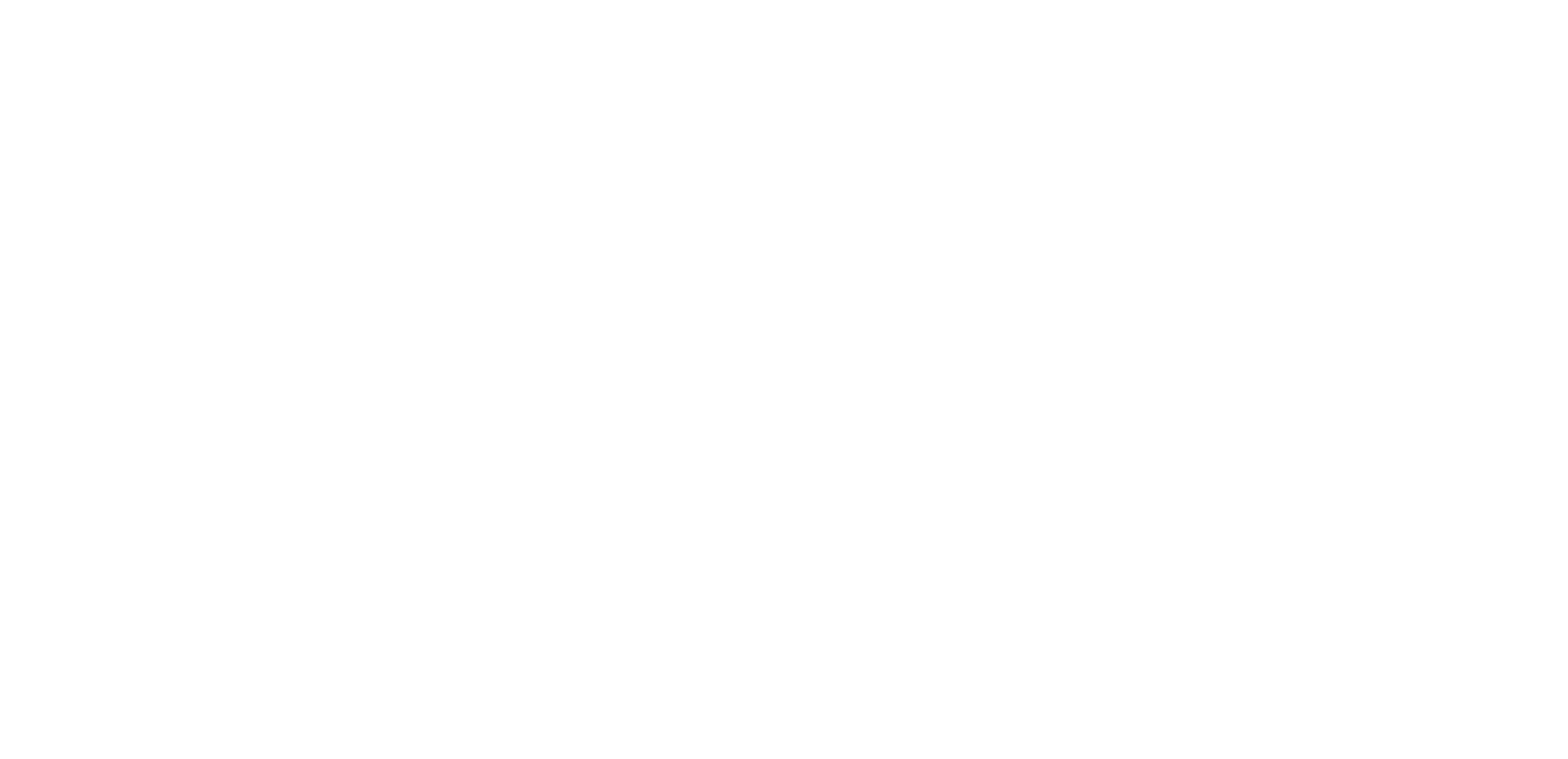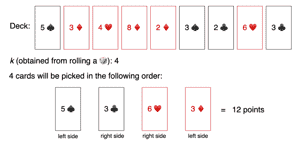In the above example, the player chose the cards 5, 3, 6, and 3 to get the maximum amount of points possible. During implementation, we’ll get a deck of cards in array form, like {5, 3, 4, 4, 2, 3, 4, 6, 3}. The number we get after rolling the dice will be given as an integer. The module should return the maximum number of points as an integer.

### Solution

To implement this feature, we need to test every possible combination in which k cards can be picked from the deck from the left or right side. We can’t pick the nth card from the right (or left), unless the (n - 1)th card from the right (or left) is picked. If we pick k - 1 cards from the right, then 1 card will be picked from the left side, and so on. We can find all possible combinations by assuming a sliding window of k size that wraps from right to left. The output will be the maximum sum found by trying all of the possible combinations.

Let’s get started coding this feature! We’ll break it down into steps. Before we get started, we need to import the proper packages so we can test our code at the end.

package main
import (
"fmt"
"math"
)

Step 1: Set up the function.

func maxPoints(deck []int, k int) int{
left := 0;
right := len(deck) - k
var total, best int
total = 0

Step 2: Assume that k cards on the right side give us the maximum points.

    for i := right; i < len(deck); i++ {
total += deck[i]
}
best = total

Step 3: Use a loop that runs k times and test all the combinations.

    for i := 0; i < k; i++ {

Step 4: Remove the points of the card on the right side and add the points on the left side.

        total += deck[left] - deck[right]

Step 5: Compare the total points with the current best points and keep the maximum.

        best = int(math.Max(float64(best), float64(total)))
left++
right++
}
return best
}

Now, let’s test our feature using a sample deck.

package main
import (
"fmt"
"math"
)
func maxPoints(deck []int, k int) int{
left := 0;
right := len(deck) - k
var total, best int
total = 0
for i := right; i < len(deck); i++ {
total += deck[i]
}
best = total
for i := 0; i < k; i++ {
total += deck[left] - deck[right]
best = int(math.Max(float64(best), float64(total)))
left++
right++
}
return best
}

func main() {
deck := []int{5,3,4,4,2,3,2,6,3}
k := 4
fmt.Println(maxPoints(deck, k))
}

## Project wrap up and related interview questions

Congrats! You made it to the end of the project. Throughout this project, we learned how to:

• Build a feature that can identify a hand of straights by grouping cards in sequential order
• Use the sliding window technique to build a feature to find cards with the maximum point values

The problems that we solved today are also commonly asked interview questions at top tech companies. After our experience building the project, you now can identify problems with the same patterns and solve those problems using the techniques we implemented today. Let’s take a look at a couple of related interview questions that we can solve using a similar approach:

• Divide an array in sets of K consecutive numbers
• Find the maximum sum from either end of an array

## Next steps

Building a strong coding portfolio gets you hands-on practice with real-world software development problems. A strong portfolio is a great way to set yourself apart from the competition as a Go developer. We completed just one project today, but there are so many more real-world projects that you could work on to learn more about the software development world and help you better prepare yourself for your Go interview. Some more project ideas include:

• Improving the user experience in finding content to watch on Netflix
• Implementing productivity-enhancing features for Google Calendar
• Optimizing customer-facing features on Amazon

To get hands-on with these projects and more, check out Educative’s course Decode the Coding Interview in Go: Real-World Examples. In this curated course, you’ll prepare for your Go interview by tackling real-world problems faced by top tech companies. After each project, you’ll learn about what kinds of interview questions are related to the project, so you’re even more prepared for your interview.

If you want to prepare for more coding interviews, check out our Decode the Coding Interview series. We have courses in the following languages:

Happy learning!

### Continue learning about coding interview projectsWRITTEN BYErin Schaffer

Join a community of more than 1 million readers. A free, bi-monthly email with a roundup of Educative's top articles and coding tips.

Learn in-demand tech skills in half the time